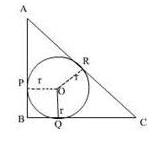MCQ: Triangle - Class 10

# MCQ: Triangle - Class 10 Notes - Class 10

## Document Description: MCQ: Triangle - Class 10 for Class 10 2022 is part of Class 10 preparation. The notes and questions for MCQ: Triangle - Class 10 have been prepared according to the Class 10 exam syllabus. Information about MCQ: Triangle - Class 10 covers topics like and MCQ: Triangle - Class 10 Example, for Class 10 2022 Exam. Find important definitions, questions, notes, meanings, examples, exercises and tests below for MCQ: Triangle - Class 10.

Introduction of MCQ: Triangle - Class 10 in English is available as part of our Class 10 preparation & MCQ: Triangle - Class 10 in Hindi for Class 10 courses. Download more important topics, notes, lectures and mock test series for Class 10 Exam by signing up for free. Class 10: MCQ: Triangle - Class 10 Notes - Class 10

ABC is a right triangle right angled at B such that BC = 6 cm and AB = 8 cm. The radius of its incircle is• a)
3 cm
• b)
8 cm
• c)
2 cm
• d)
5 cm

Ref: https://edurev.in/question/579432/ABC-is-a-right-triangle-right-angled-at-B-such-tha

 1 Crore+ students have signed up on EduRev. Have you?

Let ABC be the right angled triangle such that ∠B = 90° , BC = 6 cm, AB = 8 cm. Let O be the centre and r be the radius of the in circle.AB, BC and CA are tangents to the circle at P, N and M.

∴ OP = ON = OM = r (radius of the circle)By Pythagoras theorem,

CA2 = AB2 + BC2

⇒ CA2 = 82 + 62

⇒ CA2 = 100

⇒ CA = 10 cm

Area of ∆ABC = Area ∆OAB + Area ∆OBC + Area ∆OCAThe document MCQ: Triangle - Class 10 Notes - Class 10 is a part of Class 10 category.
All you need of Class 10 at this link: Class 10
 Use Code STAYHOME200 and get INR 200 additional OFF

Track your progress, build streaks, highlight & save important lessons and more!

,

,

,

,

,

,

,

,

,

,

,

,

,

,

,

,

,

,

,

,

,

;